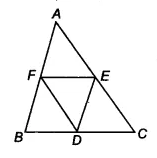# Q: 5    D, E and F are respectively the mid-points of the sides BC, CA and AB of a . Show that            (ii)We already proved that BDEF is a ||gm.
Similarly, DCEF and DEAF are also parallelograms.
Now, ||gm BDEF and ||gm DCEF is on the same base EF and between same parallels BC and EF
Ar (BDEF) = Ar (DCEF)
Ar(BDF) = Ar (DEF) .............(i)

It is known that diagonals of ||gm divides it into two triangles of equal area.
Ar(DCE) = Ar (DEF).......(ii)

and, Ar(AEF) = Ar (DEF)...........(iii)

From equation(i), (ii) and (iii), we get

Ar(BDF) =  Ar(DCE) = Ar(AEF) =  Ar (DEF)

Thus, Ar (ABC) =  Ar(BDF) +  Ar(DCE) + Ar(AEF) +  Ar (DEF)
Ar (ABC) = 4 . Ar(DEF)

Hence proved.

## Related Chapters

### Preparation Products

##### JEE Main Rank Booster 2021

This course will help student to be better prepared and study in the right direction for JEE Main..

₹ 13999/- ₹ 9999/-
##### Rank Booster NEET 2021

This course will help student to be better prepared and study in the right direction for NEET..

₹ 13999/- ₹ 9999/-
##### Knockout JEE Main April 2021 (Easy Installments)

An exhaustive E-learning program for the complete preparation of JEE Main..

₹ 4999/-
##### Knockout NEET May 2021

An exhaustive E-learning program for the complete preparation of NEET..

₹ 22999/- ₹ 14999/-